# Finding bright objects

In a blog comment earlier this summer, someone asked how to find "which labeled regions have a bright spot greater than some threshold?" That's a good question. It can be done efficiently and compactly using the Image Processing Toolbox, but the techniques may not be widely known. (Have you ever used the ismember function to do image processing before?) Also, the techniques apply to other questions of the form "which regions satisfy some criterion?"

Let's work with the rice image.

I = imread('rice.png');
imshow(I)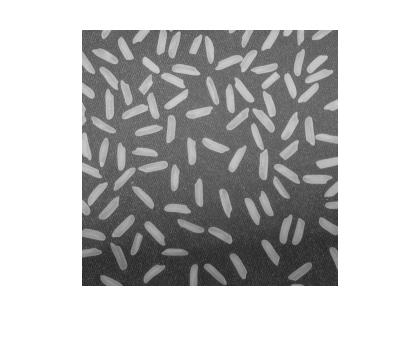Threshold it.

bw = im2bw(I, graythresh(I));
imshow(bw)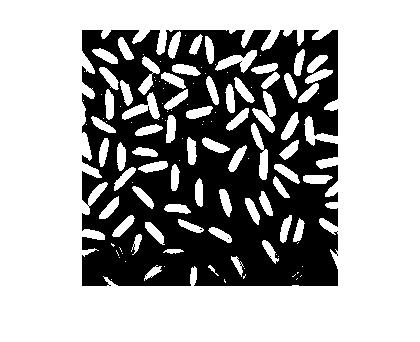Clean it up a bit.

bw = bwareaopen(bw, 50);
imshow(bw)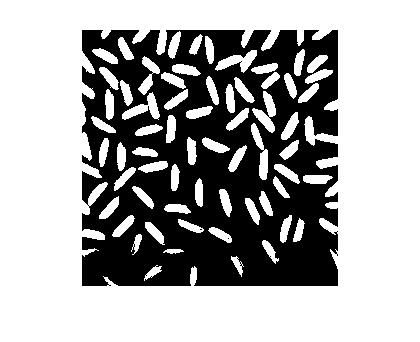Now label connected components and compute the PixelIdxList property for each labeled region. The PixelIdxList is useful for the extracting pixels from a grayscale image that correspond to each labeled region in the binary image. (See my post "Grayscale pixel values in labeled regions.")

L = bwlabel(bw);
s = regionprops(L,'PixelIdxList');

Compute the maximum pixel value for each labeled region.

% Initialize vector containing max values.
max_value = zeros(numel(s), 1);

% Loop over each labeled object, grabbing the gray scale pixel values using
% PixelIdxList and computing their maximum.
for k = 1:numel(s)
max_value(k) = max(I(s(k).PixelIdxList));
end

% Show all the maximum values as a bar chart.
bar(max_value)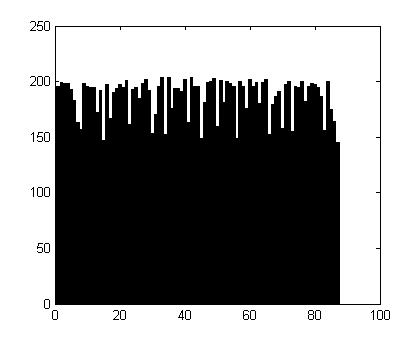And we'll finish by using find to determine which objects have a maximum value greater than 200. Then we'll display those objects using ismember.

bright_objects = find(max_value > 200)
bright_objects =

22
28
33
35
40
42
49
51
60
65


imshow(ismember(L, bright_objects))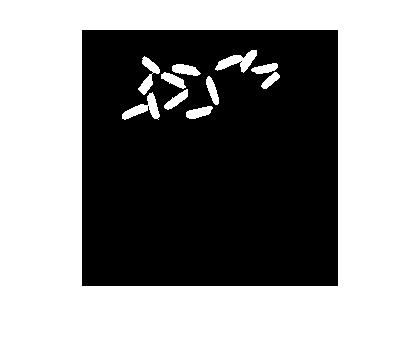Published with MATLAB® 7.5

|

### コメント

コメントを残すには、ここ をクリックして MathWorks アカウントにサインインするか新しい MathWorks アカウントを作成します。